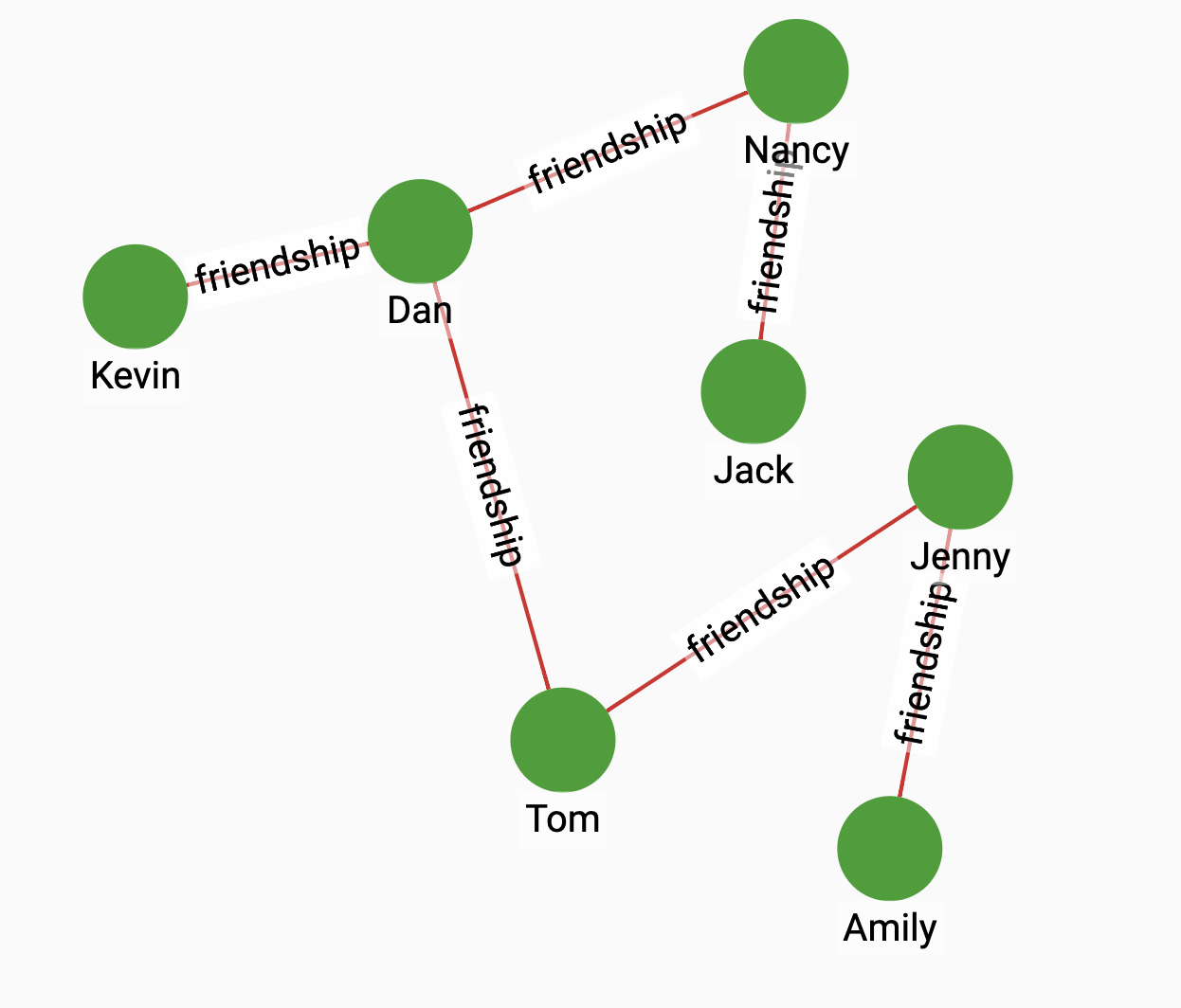# Degree Centrality

Degree centrality is defined as the number of edges incident upon a node (i.e., the number of ties that a node has). The degree can be interpreted in terms of the immediate risk of a node for catching whatever is flowing through the network (such as a virus, or some information).

## Specification

```CREATE QUERY tg_degree_cent(SET<STRING> v_type, SET<STRING> e_type,
SET<STRING> re_type, BOOL in_degree = TRUE, BOOL out_degree = TRUE,
INT top_k=100, BOOL print_accum = True, STRING result_attr = "",
STRING file_path = "")```

### Time complexity

The algorithm has a time-complexity of O(E), where E is the total number of edges in the graph.

## Parameters

Name Description Data type

`v_type`

A set of vertex types.

`SET<STRING>`

`e_type`

A set of edge types.

`SET<STRING>`

`re_type`

A set of reverse edge types. If an edge is undirected, put the edge name in the set as well.

``SET`

`in_degree`

Boolean value that indicates whether to count the incoming edges as part of a vertex’s degree centrality.

`BOOL`

`out_degree`

Boolean value that indicates whether to count the outgoing edges as part of a vertex’s degree centrality.

`BOOL`

`top_k`

The number of vertices with the highest scores to return.

`INT`

`print_accum`

If true, print results to JSON output.

`BOOL`

`result_attr`

If not empty, save the degree centrality score of each vertex to this attribute.

`STRING`

`file_attr`

If not empty, save results in CSV to this file.

`STRING`

## Return value

The vertices with the highest degree centrality scores along with their scores.

## Example

Suppose we have the following graph:Running the query on the graph will show that Dan has the highest degree centrality:

• Query

• Result

``RUN QUERY tg_degree_cent(["person"], ["friendship"],["friendship"])``
``````{
"error": false,
"message": "",
"version": {
"schema": 2,
"edition": "enterprise",
"api": "v2"
},
"results": [{"top_scores": [
{
"score": 8,
"Vertex_ID": "Dan"
},
{
"score": 6,
"Vertex_ID": "Jenny"
},
{
"score": 4,
"Vertex_ID": "Nancy"
},
{
"score": 2,
"Vertex_ID": "Kevin"
},
{
"score": 2,
"Vertex_ID": "Amily"
},
{
"score": 2,
"Vertex_ID": "Jack"
}
]}]
}``````